Diffusion

Diffusion is the process behind Brownian motion. It was described by Albert Einstein in one of his annus mirabilis (1905) papers. The diffusion equation is that describes the process is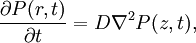$\frac{\partial P(r,t)}{\partial t}= D \nabla^2 P(z,t),$

where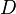$D$ is the (self-)diffusion coefficient. For initial conditions for a Dirac delta function at the origin, and boundary conditions that force the vanishing of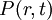$P(r,t)$ and its gradient at large distances, the solution factorizes as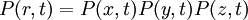$P(r,t)=P(x,t)P(y,t)P(z,t)$, with a spreading Gaussian for each of the Cartesian components: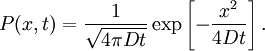$P(x,t)=\frac{1}{\sqrt{4\pi D t}} \exp \left[ - \frac{x^2}{4 D t} \right].$

Einstein relation

For a homogeneous system,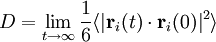$D = \lim_{t \rightarrow \infty} \frac{1}{6} \langle \vert \mathbf{r}_i(t) \cdot \mathbf{r}_i(0) \vert^2\rangle$

Green-Kubo relation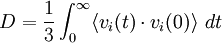$D = \frac{1}{3} \int_0^\infty \langle v_i(t) \cdot v_i(0)\rangle ~dt$

where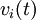$v_i(t)$ is the center of mass velovity of molecule$i$.